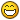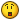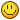# Random path Walking (use waypoints)

## Recommended Posts

(Apparently this forums do not like to keep my tabbed code)

This code will walk a random path every time to a coordinate, use waypoints to move around buildings/fences

will return true once it is within 5 spaces of the end point.

(WILL WALK ANY DISTANCE)

tabbed version- http://pastebin.com/djSDJb2p

```public long lastRun = 0;
public Position lastClick = null;
public int randomDistance = 0;

public boolean walkToLocation(Position p) throws InterruptedException {
int maxY;
int maxX;
boolean revY = false;
boolean revX = false;
Position playerPosition = myPlayer().getPosition();
if(playerPosition.getX() < p.getX()) {
revX = true;
int distance = p.getX()-playerPosition.getX();
if(distance > 17) {
maxX = 17;
} else {
maxX = distance;
}
} else {
int distance = playerPosition.getX()-p.getX();
if(distance > 17) {
maxX = 17;
} else {
maxX = distance;
}
}
if(playerPosition.getY() < p.getY()) {
revY = true;
int distance = p.getY()-playerPosition.getY();
if(distance > 17) {
maxY = 17;
} else {
maxY = distance;
}
} else {
int distance = playerPosition.getY()-p.getY();
if(distance > 17) {
maxY = 17;
} else {
maxY = distance;
}
}
if(maxX < 10) {
}
if(maxY < 10) {
}
if(!revX) {
}
if(!revY) {
}
if(lastClick == null) {
randomDistance = (int) (3+(Math.random() * 3));
}
} else {
if(lastClick.distance(myPlayer().getPosition()) < randomDistance) {
randomDistance = (int) (3+(Math.random() * 3));
}
}
}
if(lastClick.distance(p) < 5) {
lastClick = null;
return true;
}
return false;
}

```

Use (in the onLoop() Method)

```if(walkToLocation(new Position(x,y,z))) {
//what happens once it reaches end
}
```

Will release a detailed guide soon to determine waypoints

Guide:AndEdited by LifezHatred

##### Share on other sites

Looks nice! Is there a possibility to make a script purely for walking? The otherone thats currently online doesn't work. I'm sure this one will work##### Share on other sites

Looks nice! Is there a possibility to make a script purely for walking? The otherone thats currently online doesn't work. I'm sure this one will worki guess i could add a few more methods to it, n put it in a class

the only thing it would need now is add a a method that executes waypoints

which would be something like

```public Position[] waypoints = {...};
public int offset = 0;

public boolean executeWaypoints(Position[] p) {
if(walkToLocation(p[offset])) {
offset++;
if(offset == p.length) {
offset = 0;
return true;
}
}
return false;
}
```

which returns true once it reaches the end

Edited by LifezHatred

##### Share on other sites

Never realized how much I love the tab key until I saw thisBut anyway it looks nice, I'll work this into one of my scripts somewhere!

##### Share on other sites

Never realized how much I love the tab key until I saw thisBut anyway it looks nice, I'll work this into one of my scripts somewhere!

yeah apparently no matter how many times i re pasted it the tabs would not show up =[

tabbed version - http://pastebin.com/djSDJb2p

Edited by LifezHatred

##### Share on other sites

Never realized how much I love the tab key until I saw thisBut anyway it looks nice, I'll work this into one of my scripts somewhere!

yeah apparently no matter how many times i re pasted it the tabs would not show up =[

tabbed version - http://pastebin.com/djSDJb2p

You have to use spaces instead of tabs.

Also the word wrapping can mess things up so, start any new line on a new line to prevent weird word wrapping.

Hope you don't mind here is the code formatted below```public long lastRun = 0;
public Position lastClick = null;
public int randomDistance = 0;

public boolean walkToLocation(Position p) throws InterruptedException
{
int maxY;
int maxX;
boolean revY = false;
boolean revX = false;
Position playerPosition = myPlayer().getPosition();
if(playerPosition.getX() < p.getX())
{
revX = true;
int distance = p.getX()-playerPosition.getX();
if(distance > 17)
{
maxX = 17;
}
else
{
maxX = distance;
}
}
else
{
int distance = playerPosition.getX()-p.getX();
if(distance > 17)
{
maxX = 17;
}
else
{
maxX = distance;
}
}
if(playerPosition.getY() < p.getY())
{
revY = true;
int distance = p.getY()-playerPosition.getY();
if(distance > 17)
{
maxY = 17;
}
else
{
maxY = distance;
}
}
else
{
int distance = playerPosition.getY()-p.getY();
if(distance > 17)
{
maxY = 17;
}
else
{
maxY = distance;
}
}
if(maxX < 10)
{
}
if(maxY < 10)
{
}
if(!revX)
{
}
if(!revY)
{
}
if(lastClick == null)
{
{
randomDistance = (int) (3+(Math.random() * 3));
}
}
else
{
if(lastClick.distance(myPlayer().getPosition()) < randomDistance)
{
{
randomDistance = (int) (3+(Math.random() * 3));
}
}
}
if(lastClick.distance(p) < 5)
{
lastClick = null;
return true;
}
return false;
}
```

##### Share on other sites

Never realized how much I love the tab key until I saw thisBut anyway it looks nice, I'll work this into one of my scripts somewhere!

yeah apparently no matter how many times i re pasted it the tabs would not show up =[

tabbed version - http://pastebin.com/djSDJb2p

You have to use spaces instead of tabs.

Also the word wrapping can mess things up so, start any new line on a new line to prevent weird word wrapping.

Hope you don't mind here is the code formatted below```public long lastRun = 0;
public Position lastClick = null;
public int randomDistance = 0;

public boolean walkToLocation(Position p) throws InterruptedException
{
int maxY;
int maxX;
boolean revY = false;
boolean revX = false;
Position playerPosition = myPlayer().getPosition();
if(playerPosition.getX() < p.getX())
{
revX = true;
int distance = p.getX()-playerPosition.getX();
if(distance > 17)
{
maxX = 17;
}
else
{
maxX = distance;
}
}
else
{
int distance = playerPosition.getX()-p.getX();
if(distance > 17)
{
maxX = 17;
}
else
{
maxX = distance;
}
}
if(playerPosition.getY() < p.getY())
{
revY = true;
int distance = p.getY()-playerPosition.getY();
if(distance > 17)
{
maxY = 17;
}
else
{
maxY = distance;
}
}
else
{
int distance = playerPosition.getY()-p.getY();
if(distance > 17)
{
maxY = 17;
}
else
{
maxY = distance;
}
}
if(maxX < 10)
{
}
if(maxY < 10)
{
}
if(!revX)
{
}
if(!revY)
{
}
if(lastClick == null)
{
{
randomDistance = (int) (3+(Math.random() * 3));
}
}
else
{
if(lastClick.distance(myPlayer().getPosition()) < randomDistance)
{
{
randomDistance = (int) (3+(Math.random() * 3));
}
}
}
if(lastClick.distance(p) < 5)
{
lastClick = null;
return true;
}
return false;
}

```

i like my conventions better, yours is more of a C++ convention

plus if people want a tabbed version i did provide a link

Edited by LifezHatred

##### Share on other sites

Thank you for this. Been working on a project that doesnt cope well with the usual walking methods and this saved me alot of head ache. Thanks##### Share on other sites

Thank you for this. Been working on a project that doesnt cope well with the usual walking methods and this saved me alot of head ache. ThanksNp man, its less detectable because it uses a different path to that location every time (in my yew cutter i released it never runs the same path)

Edited by LifezHatred

##### Share on other sites

This looks awesome. I'll wait for the more detailed guide, thanks for sharing!

##### Share on other sites

Perhaps you should simplify your code to something like:

```public static Position getNextPosition(Script script, Position end) {

Position  cur = script.myPosition();

int disX = end.getX() - cur.getX(), disY = end.getY() - cur.getY();
boolean revX = disX < 0, revY = disY < 0;

disX = Math.abs(disX); disY = Math.abs(disY);
if (disX >= 17)
disX = 10 + MethodProvider.random(5);
if (disY >= 17)
disY = 10 + MethodProvider.random(5);

return new Position(cur.getX() + (revX ? ~disX : disX), cur.getY() + (revY ? ~disY : disY), cur.getZ());
}
```

I made my method a 'getter' to allow users to provide users with the ability to handle the actual walking themselves.

Edited by liverare

##### Share on other sites

Perhaps you should simplify your code to something like:

```public static Position getNextPosition(Script script, Position end) {

Position  cur = script.myPosition();

int disX = end.getX() - cur.getX(), disY = end.getY() - cur.getY();
boolean revX = disX < 0, revY = disY < 0;

disX = Math.abs(disX); disY = Math.abs(disY);
if (disX >= 17)
disX = 10 + MethodProvider.random(5);
if (disY >= 17)
disY = 10 + MethodProvider.random(5);

return new Position(cur.getX() + (revX ? ~disX : disX), cur.getY() + (revY ? ~disY : disY), cur.getZ());
}
```

I made it a getter method because I assume people will want to provide better structured handling for walking--instead of trusting this method will get them from A to B.

Ehh i guess, but i'd rather keep mine the way it is

##### Share on other sites

. . .

Ehh i guess, but i'd rather keep mine the way it is

Why? Your code is 74 lines long (2.34 KB) whereas mine is only 11 lines long (522 bytes). They both draw pretty much the same conclusion aside from mine lacking the walking handler. Program however you want, but condensing code whilst maintaining its overarching functionality and purpose is good practice. It helps remove redundant lines which help improve readability.

[EDIT] I should also note that Pork has produced a much better method than both of ours because his uses some nice mathematics.

Edited by liverare
•1

##### Share on other sites

. . .

Ehh i guess, but i'd rather keep mine the way it is

Why? Your code is 74 lines long (2.34 KB) -- most of which is redundant. Mine is 11 (522 bytes) and it draws pretty much the same conclusion (without walking handler). I'm not going to tell you how to program, but at least have consideration for possible improvements.

because idc bout all that stuff?

don't post on my thread anymore if all ur going to do is complain about what i do

##### Share on other sites

Thanks for the snippet.

Also liverare was trying to improve ya code no need to go angry on the guy :p